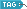## uint8, uint16, uint32, uint64

: 2009. 9. 8. 15:43반응형
Convert to unsigned integer

Syntax

• ```i = uint8(x)
i = uint16(x)
i = uint32(x)
i = uint64(x)
```

Description

`i = uint*(x) `converts the vector `x` into an unsigned integer. `x` can be any numeric object (such as a `double`). The results of a `uint*` operation are shown in the next table.

 Operation Output Range Output Type Bytes per Element Output Class `uint8` 0 to 255 Unsigned 8-bit integer 1 `uint8` `uint16` 0 to 65,535 Unsigned 16-bit integer 2 `uint16` `uint32` 0 to 4,294,967,295 Unsigned 32-bit integer 4 `uint32` `uint64` 0 to 18,446,744,073,709,551,615 Unsigned 64-bit integer 8 `uint64`

A value of `x` above or below the range for a class is mapped to one of the endpoints of the range. If `x` is already an unsigned integer of the same class, `uint*` has no effect.

The `uint*` class is primarily meant to store integer values. Most operations that manipulate arrays without changing their elements are defined. (Examples are `reshape`, `size`, the logical and relational operators, subscripted assignment, and subscripted reference.) No math operations except for `sum` are defined for `uint*` since such operations are ambiguous on the boundary of the set. (For example they could wrap or truncate there.) You can define your own methods for `uint*` (as you can for any object) by placing the appropriately named method in an `@uint*` directory within a directory on your path.

Type `help datatypes` for the names of the methods you can overload.

`double`, `int8`, `int16`, `int32`, `int64`, `single`